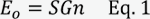### Question

Question: I want to perform a shunt calibration on my Endevco® model 7264C series piezoresistive shock accelerometers. Can you explain the basics of how to do this properly? I'm told that shunt calibration is not a ""true"" calibration.

Shunt calibration is a passive calibration, in that the accelerometer is not mechanically stimulated during the calibration process itself. This is in contrast to a dynamic calibration, in which the accelerometer is mechanically stimulated by a known amount, usually with a shaker, within a laboratory environment. Shunt calibration is usually performed after the accelerometer is installed on a test structure, making dynamic calibration impossible. A shunt calibration provides a valuable continuity check of the entire measurement chain, from transducer to data acquisition system, while providing a reliable means of verifying signal conditioner gain settings before a test is performed.

During a typical piezoresistive accelerometer shunt calibration, an accurate and stable fixed resistor is connected across one of the transducer bridge resistors (see fig. 1). This shunt resistor will unbalance the bridge and electrically simulate a pre-calculated percentage of transducer full-scale output. Thus, the accelerometer output under shunt calibration appears as if the sensor was being mechanically stimulated.

Although shunt calibration can be performed on transducers with four active arms (such as Endevco's piezoresistive pressure sensors) in the bridge, Endevco does not recommend this practice. Calculating the value of the shunt resistor requires that at least one bridge resistor value is already known. As piezoresistive sensing elements are sensitive to temperature, their resistance values will change. It is for this reason that the model 7264C series utilizes only two active arms in the bridge, while the remaining two elements are fixed, accurate resistors with a low temperature coefficient (e.g., 7264C series fixed resistors are 500 ohms Â±1% each).

Calculating the shunt resistor value is straightforward. The voltage output, due to the shunt resistor addition to the bridge, is given by:Where:

• S = sensitivity of the accelerometer (in V/g)
• G = accelerometer full-scale acceleration (in g)
• n = percentage of accelerometer full-scale output to be simulated (in decimal form)

The shunt resistor value can now be calculated using this equation:Where:

• R = value of fixed resistor in bridge (in ohms)
• EX = excitation voltage used on the bridge (in volts)
• EO = output voltage, due to the addition of the shunt resistor (calculated in Eq. 1 above)

As an example, let's assume we want to shunt calibrate an EndevcoÂ® model 7264C-2000. To calculate the shunt resistor value, we need to first know the accelerometer's sensitivity. The calibration certificate that is shipped along with the unit indicates a sensitivity of 0.19 mV/g (with a 10 Vdc excitation on the bridge). We also want to simulate 25% of accelerometer full-scale output once the shunt resistor is installed.

Using Eq. 1 above, the voltage change will be:We know from the datasheet for this particular model that the fixed resistor value for the model 7264C is 500 ohms. Using Eq. 2, we can calculate the shunt resistor value: